# 听说你还在写双层 for 循环解两数之和？

• 2021 年 11 月 13 日
• 本文字数：3004 字

阅读完需：约 10 分钟### 一、写在前面

• 初衷想刷 leetcode 也不是一两天的事情了，之前也有很多人给过建议，于是乎，就给安排上了，一来算法的确是很重要的一块，需要好好学，为了提升自己，再者，这也可以作为掘金分享的一块，给大家分享，当然，最重要的是这个过程中会结交到很多志趣相投，有想法的朋友。

• 安排目前打算一个星期刷 2-3 个题，推文分享进度可能会慢一点，但一个星期至少也会有两篇，期待大家参与。

### 二、今日题目

给定 nums = [2, 7, 11, 15], target = 9因为 nums + nums = 2 + 7 = 9所以返回 [0, 1]

### 四、解题

• 方法一：又蠢又笨的双重 for 循环

class Solution(object):    def twoSum(self, nums, target):        """        :type nums: List[int]        :type target: int        :rtype: List[int]        """        l = len(nums)        for a in range(l):            for b in range(l):                if a != b:                    if nums[a]+nums[b] == target:                        return [a,b]nums = [2, 7, 11, 15]target = 9test_o = Solution()result = test_o.twoSum(nums,target)print(result)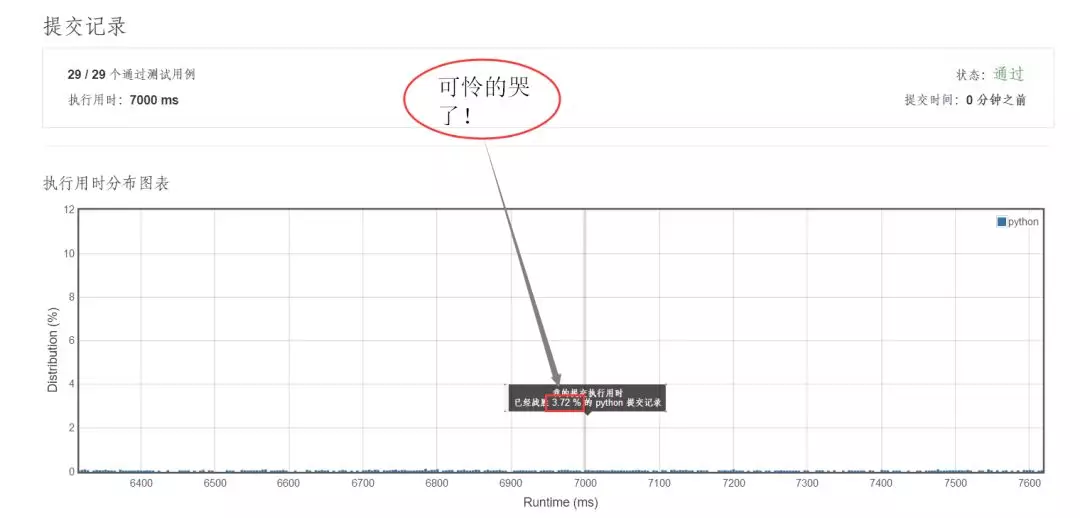• 方法二：比双 for 聪明一点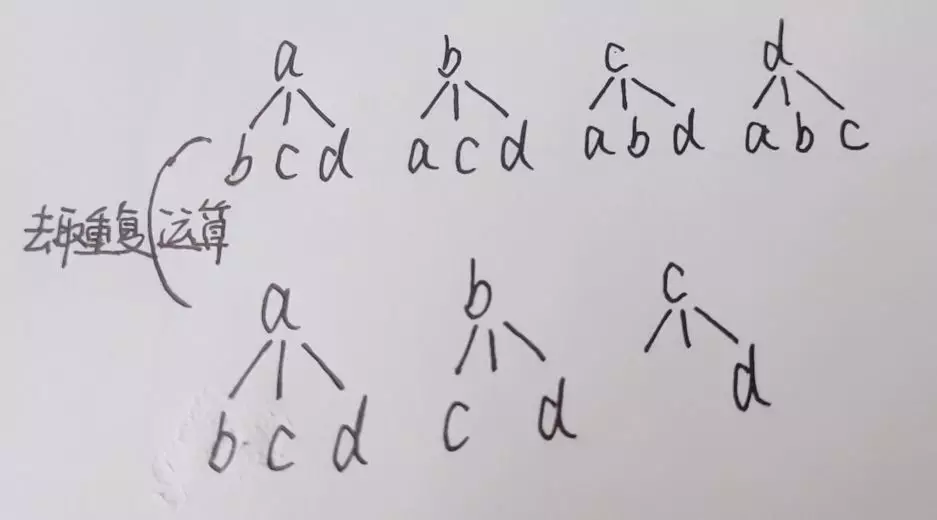class Solution(object):    def twoSum(self, nums, target):        """        :type nums: List[int]        :type target: int        :rtype: List[int]        """        flags = [0, 0, 0, 0]        l = len(nums)        for a in range(l):            flags[a]=1            for b in range(l):                if flags[b]==0:                    if nums[a]+nums[b] == target:                        return [a,b]        # 另一种简单方法                     # l = len(nums)        # for a in range(l):        #    for b in range(a+1,l):        #       if nums[a] + nums[b] == target:        #            return [a, b]

• 提交结果：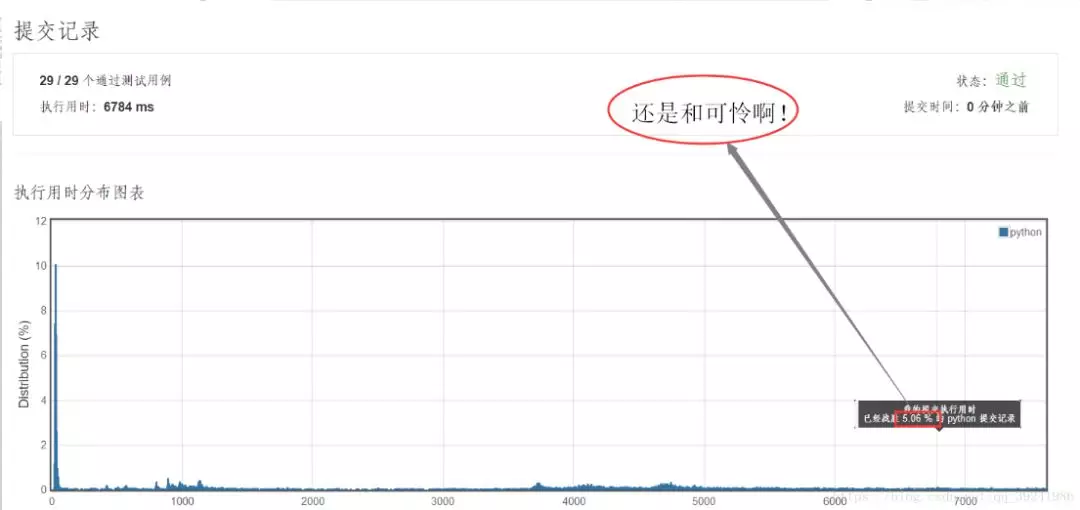• 方法二运行结果

• 方法三 ：一层循环（偷瞄了小詹学长的方法）

class Solution(object):    def twoSum(self, nums, target):        """        :type nums: List[int]        :type target: int        :rtype: List[int]        """       #flags = [0 for i in range(len(nums))]        l = len(nums)        for a in range(l):            one_unm = nums[a]            other_one = target - one_unm            if other_one in nums:                b = nums.index(other_one)                if a != b:                    if a>b:                        return [b,a]                    return [a,b]

• 提交结果：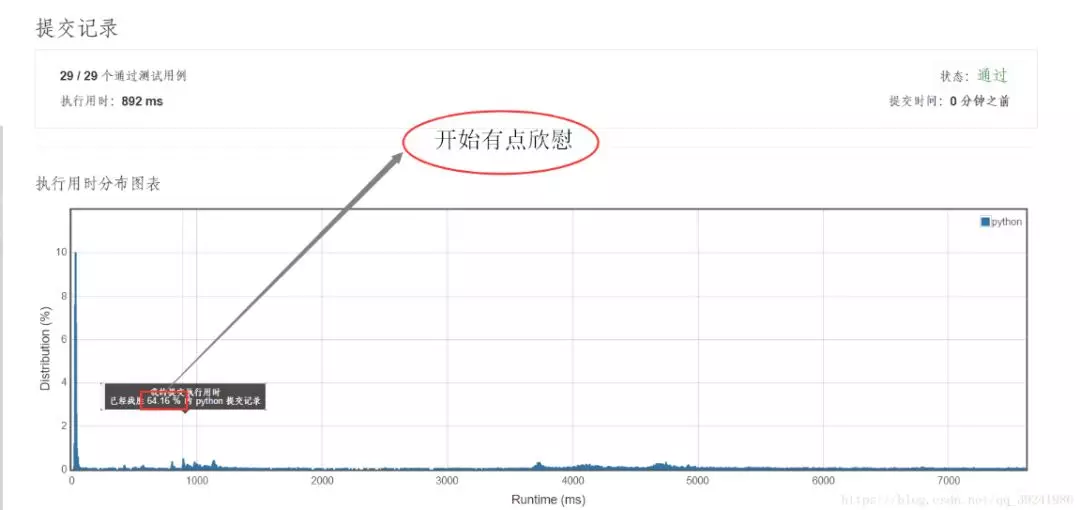• 方法三运行结果

• 方法四：一层 for 循环优化（小詹读者【村前河水流】提供）

class Solution(object):    def twoSum(self, nums, target):        """        :type nums: List[int]        :type target: int        :rtype: List[int]        """       #flags = [0 for i in range(len(nums))]        l = len(nums)        dict_nums = {nums[i]:i for i in range(l)}        for a in range(l):            one_unm = nums[a]            other_one = target - one_unm            if other_one in dict_nums and a!= dict_nums[other_one]:                return [a,dict_nums[other_one]]

• 提交结果：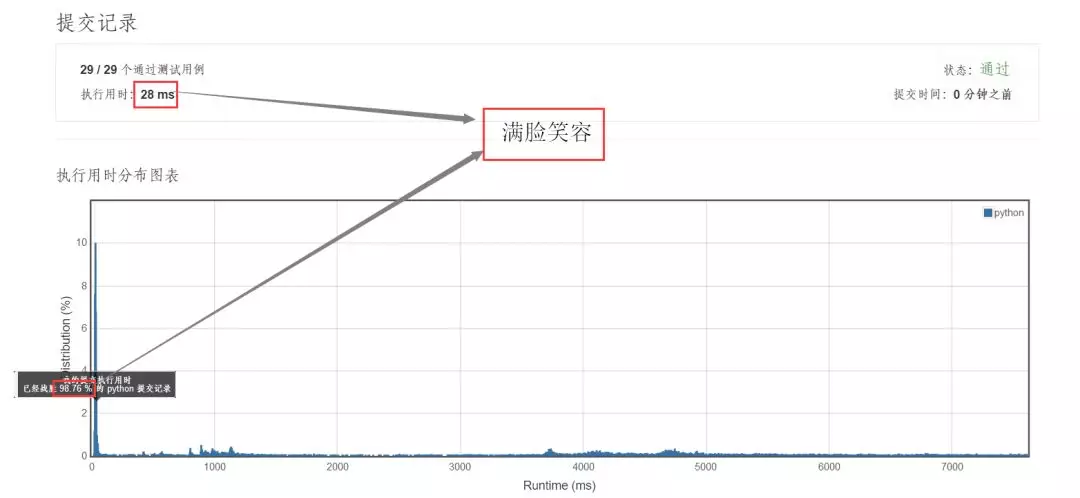• 方法四运行结果

### 五、疑惑

• 列表与字典遍历性能测试代码：

import timelist_01 = [str(i) for i in range(1000)]start_time = time.time()if 'a' in list_01 :    a = 0end_time = time.time()print("列表遍历耗时："+str(end_time-start_time))dict_01 = {str(i):i for i in range(1000)}start_time2 = time.time()if 'a' in dict_01 :    a = 0end_time2 = time.time()print("字典遍历耗时："+str(end_time2-start_time2))

• 测试结果：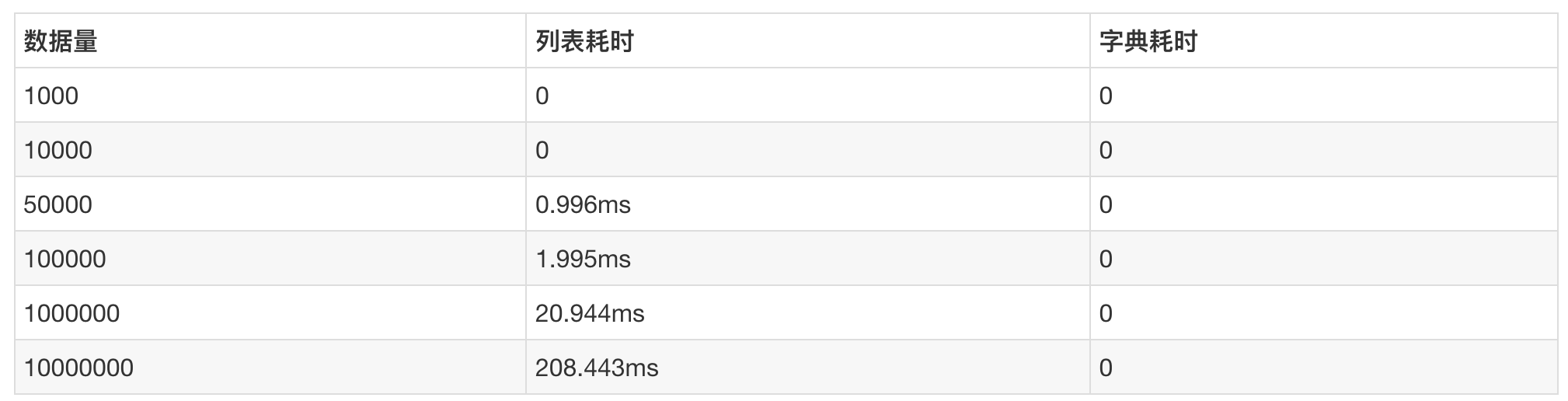### 六、结语

—《老表打油诗》### 老表

【公众号：简说Python】爱猫爱技术，Python终身学习者、数据分析爱好者、Go语言内卷机。

## 评论